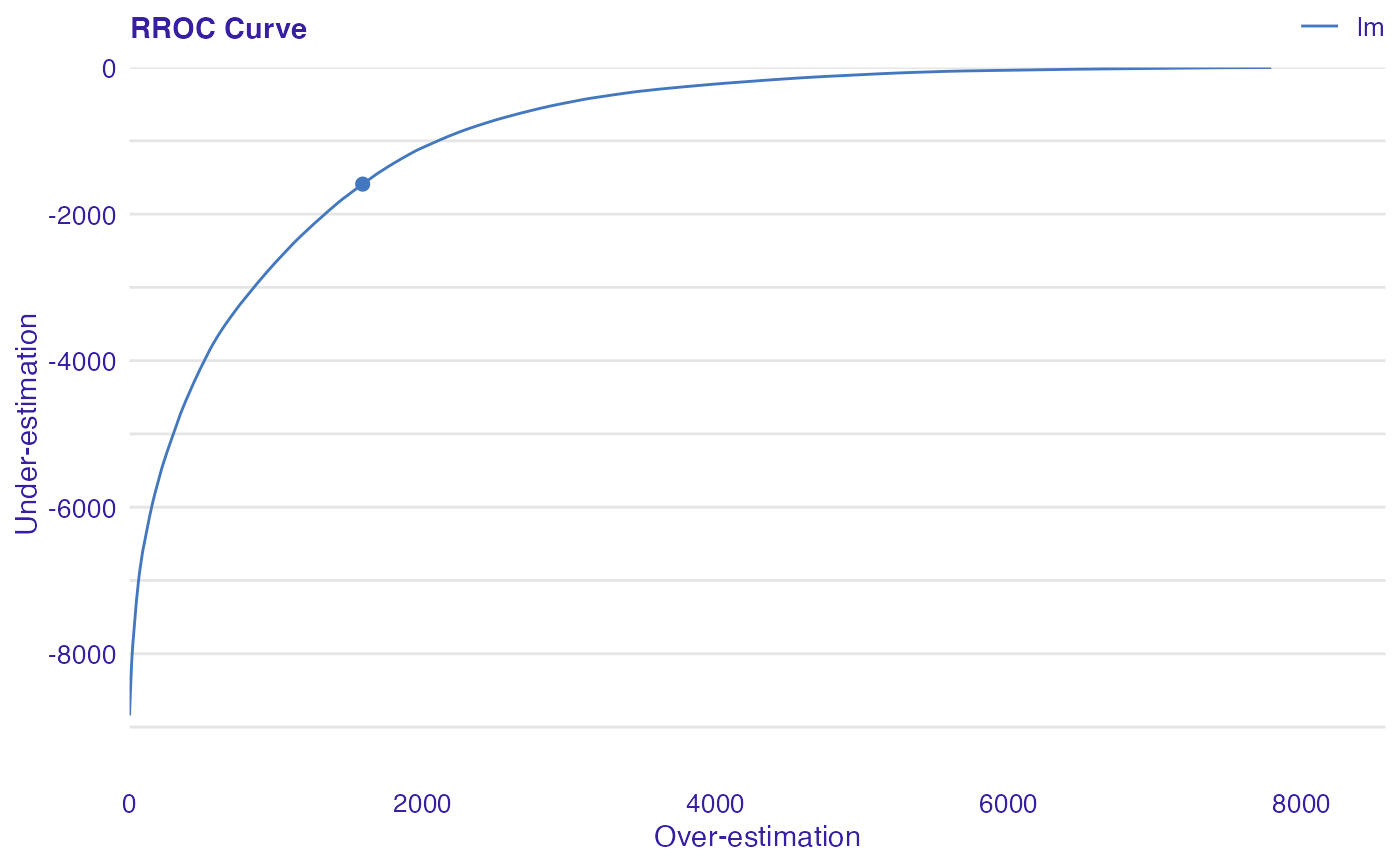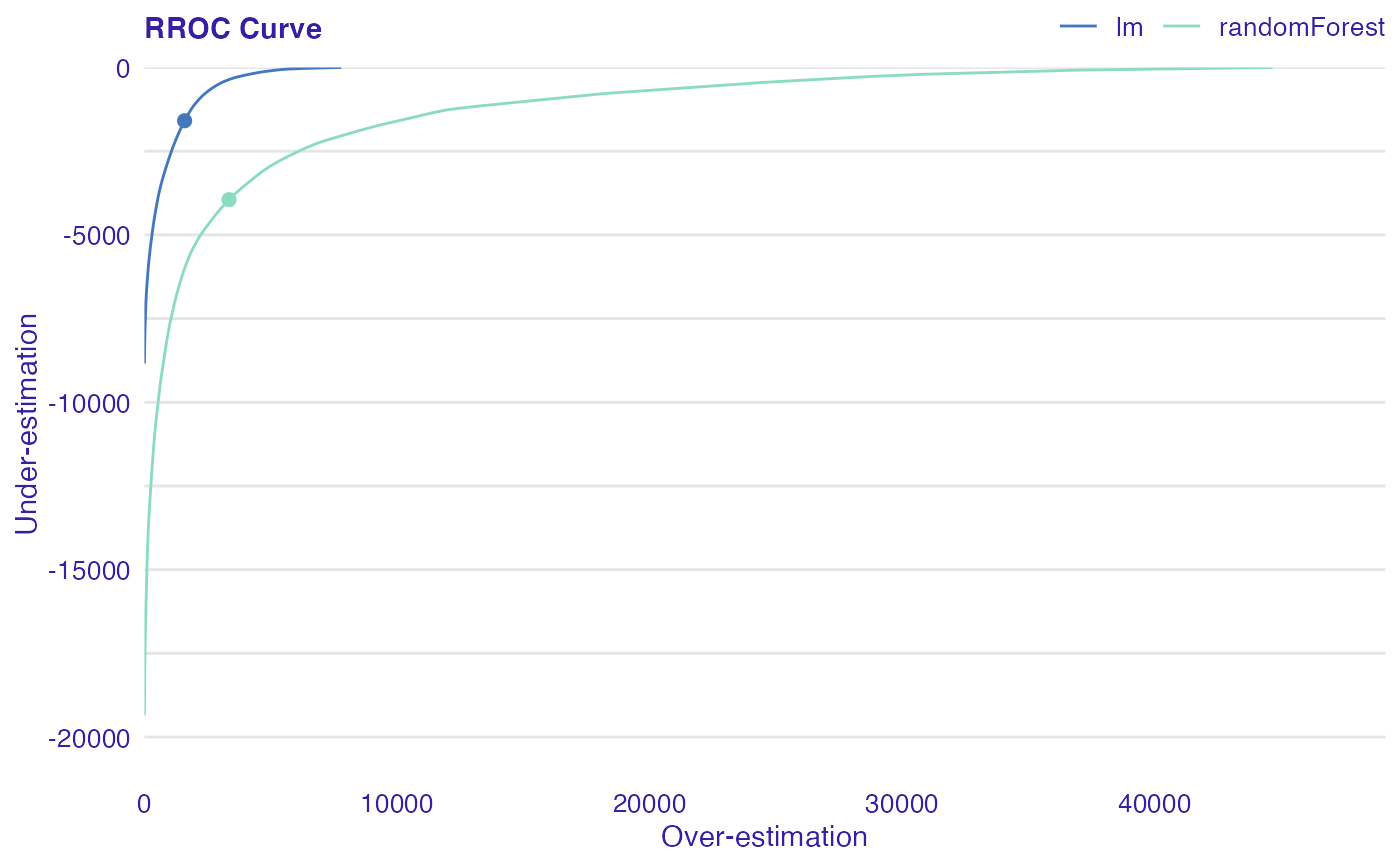The basic idea of the ROC curves for regression is to show model asymmetry. The RROC is a plot where on the x-axis we depict total over-estimation and on the y-axis total under-estimation.

plot_rroc(object, ...)

plotRROC(object, ...)

## Arguments

object An object of class auditor_model_residual created with model_residual function. Other auditor_model_residual objects to be plotted together.

A ggplot object.

## Details

For RROC curves we use a shift, which is an equivalent to the threshold for ROC curves. For each observation we calculate new prediction: $$\hat{y}'=\hat{y}+s$$ where s is the shift. Therefore, there are different error values for each shift: $$e_i = \hat{y_i}' - y_i$$

Over-estimation is calculated as: $$OVER= \sum(e_i|e_i>0)$$.

Under-estimation is calculated as: $$UNDER = \sum(e_i|e_i<0)$$.

The shift equals 0 is represented by a dot.

The Area Over the RROC Curve (AOC) equals to the variance of the errors multiplied by $$frac{n^2}{2}$$.

Hernández-Orallo, José. 2013. "ROC Curves for Regression". Pattern Recognition 46 (12): 3395–3411.

 plot_roc, plot_rec

## Examples

dragons <- DALEX::dragons[1:100, ]

# fit a model
model_lm <- lm(life_length ~ ., data = dragons)

lm_audit <- audit(model_lm, data = dragons, y = dragons$life_length) #> Preparation of a new explainer is initiated #> -> model label : lm ( default ) #> -> data : 100 rows 8 cols #> -> target variable : 100 values #> -> predict function : yhat.lm will be used ( default ) #> -> predicted values : No value for predict function target column. ( default ) #> -> model_info : package stats , ver. 4.1.1 , task regression ( default ) #> -> predicted values : numerical, min = 585.8311 , mean = 1347.787 , max = 2942.307 #> -> residual function : difference between y and yhat ( default ) #> -> residuals : numerical, min = -88.41755 , mean = -1.489291e-13 , max = 77.92805 #> A new explainer has been created! # validate a model with auditor mr_lm <- model_residual(lm_audit) # plot results plot_rroc(mr_lm)plot(mr_lm, type = "rroc")library(randomForest) model_rf <- randomForest(life_length~., data = dragons) rf_audit <- audit(model_rf, data = dragons, y = dragons$life_length)
#> Preparation of a new explainer is initiated
#>   -> model label       :  randomForest  (  default  )
#>   -> data              :  100  rows  8  cols
#>   -> target variable   :  100  values
#>   -> predict function  :  yhat.randomForest  will be used (  default  )
#>   -> predicted values  :  No value for predict function target column. (  default  )
#>   -> model_info        :  package randomForest , ver. 4.6.14 , task regression (  default  )
#>   -> predicted values  :  numerical, min =  753.2444 , mean =  1341.802 , max =  2445.824
#>   -> residual function :  difference between y and yhat (  default  )
#>   -> residuals         :  numerical, min =  -187.5791 , mean =  5.984587 , max =  452.5971
#>   A new explainer has been created!  mr_rf <- model_residual(rf_audit)
plot_rroc(mr_lm, mr_rf)plot(mr_lm, mr_rf, type="rroc")